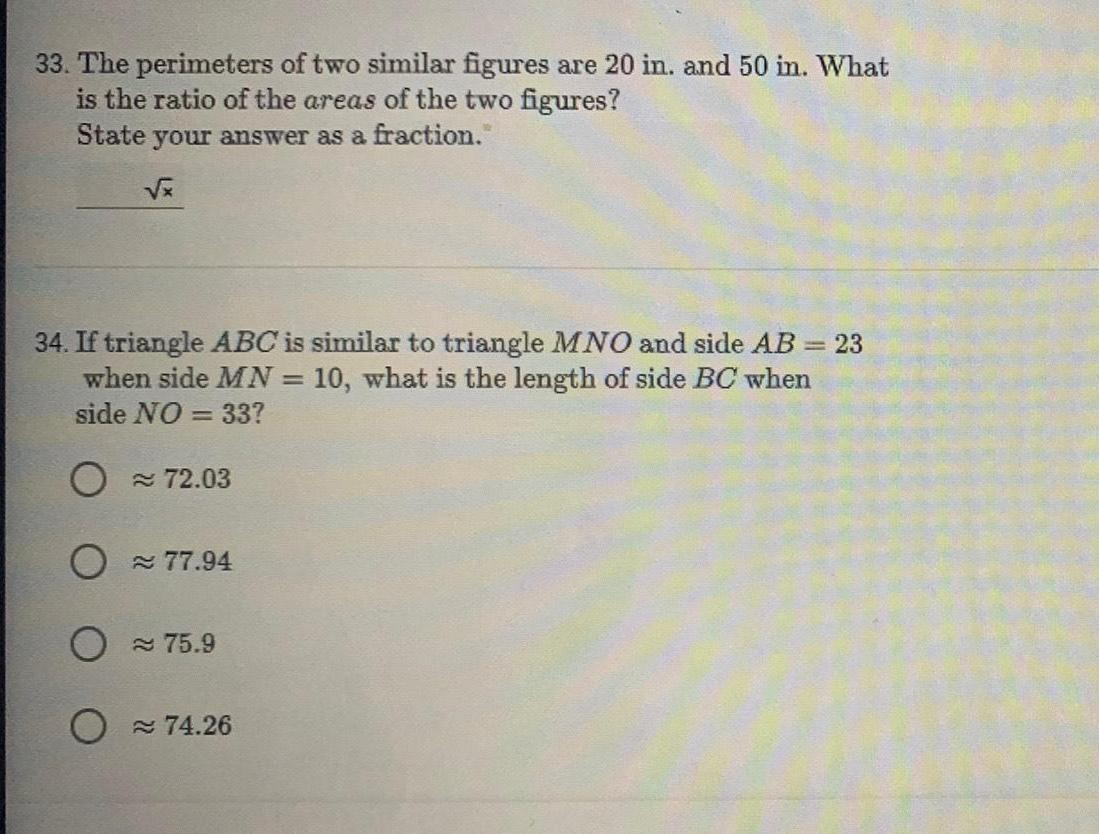Question:

# 33 The perimeters of two similar figures are 20 in and 50 in

Last updated: 9/10/202333 The perimeters of two similar figures are 20 in and 50 in What is the ratio of the areas of the two figures State your answer as a fraction 34 If triangle ABC is similar to triangle MNO and side AB 23 when side MN 10 what is the length of side BC when side NO 33 O 72 03 O 77 94 O 75 9 O 74 26